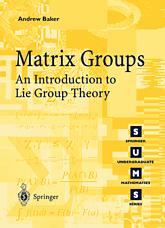## Some related books

This page contains corrections and comments for the bookMatrix Groups: An Introduction to Lie Group Theory published by Springer-Verlag. Please send me any observations, corrections, etc. using the following form and I will endeavour to publish a suitable response.

### Corrections to the second printing

Page 68, line 3: the Jacobi identity should be

[x,[y,z]]+[y,[z,x]]+[z,[x,y]]=0.

Page 106, Prop. 4.21: u statement should end with ...and each Ae_i is a simple algebra.'.

Page 140, line 1: u should be an element of Gamma_n.

### Corrections to the first printing

#### Page vii, Some suggestions for reading this book

Line 4: Chapters 1,3,7' should be Chapters 1,2,3,7'.

#### Page 6, line 8

Second M_n(R)' should be M_n(C)'.

#### Page 12, section 1.3, line 4:

domain' should be codomain'.

#### Page 111, line 14 after Defn 4.31:

nu(a,b)<=nu(a)nu(b)' should be nu(a,b)=nu(a)nu(b)''.

#### Page 114, Prop 4.37, 2nd equation:

The sum on the left side should be from 1 to m, not 1 to n, and the sum of the right side should be over r not s. In the next equation, the x's should go from 1 to m.

#### Page 119, line 6:

... k = i = -kj ...' should be ... jk = i = -kj ...'.

#### Page 120, line 14:

z=' should be z=u1 + vi'.

#### Page 125, Example 4.52, line 7:

The middle equation should read alpha(j)=Aj'.

#### Page 128, Question 4.9, line 2:

The equation should read ...=dim Symp_{2n}(R)'.

#### Page 130, equation (5.1)

e_se_r = -e_se_r' should be e_se_r = -e_re_s'.

#### Page 134, line -7:

The second =' is missing.

#### Page 163, Proposition 6.6

U_k=...' should be U_j=...'.

In the equation for <U_j,U_k>, the last case should read if j=k=n+1'.

#### Page 165, line -7:

The 2 times 2 matrix should be R(theta) (with no subscript).

#### Page 169, line -3:

In the definition of U'', the factor in front should be 1/2i'.

#### Page 171, Section 6.3, line 1:

Should read the Lie algebra of'.

#### Page 182, Definition 7.2:

This should read A topological space X is separable if it has a countable basis, i.e., a basis of the form...'.

#### Page 182, Definition 7.3, line 4:

Should read ...a collection of n-charts'.

#### line -10:

The domain of f_0 circ gamma' should be(a,b) cap gamma^{-1}f_0^{-1}V_0'.

#### line -7:

The domain of f_0 circ gamma' should be (a,b) cap gamma^{-1}f^{-1}V_0'.

#### Page 184, line 7:

(f\gamma)'(0)' should be (f_0\gamma)'(0)'.

#### Page 193, lines -9, -7, -5:

In each case, the sums should be over k=>2' rather than k<=2'.

#### Page194, line 14

1/2||C||^2 should be 1/2||C||.

#### Page 205, line -3, -1:

In each case the second vector should be (0,1,0)'.

#### Page 206, line -9:

Should read ...which shows that phi(g) v is another'.

#### Page 206, line -2:

Should read ...there are non-zero vectors'.

#### Page 212, line -14:

pi U subset G' should be pi U subset G/H'.

#### Page 213, diagram:

The right hand arrow should be labelled with f' and the bottom arrow with overline{f}'.

#### Page 242, line 7:

p(0)=x and q(0)=y' should be p(1)=x and q(1)=y'.

Page 308, line -1:

The (2,1) entry -2n' in the matrix on the right hand side should be `-2nt^{n-1}'.

#### Principal vs Principle

Yes I know, Principle in Theorems 10.13 & 10.15 (and anywhere else) should be Principal.

### Thanks

Thanks to R. Barraclough, R. Chapman, P. Eccles, Robin Endelman, S. Hendren, N. Pollock, M. Yankelevitch and R. Wickner for spotting the above.

 Last updated on   6th November 2007 Page maintained by   Andrew Baker